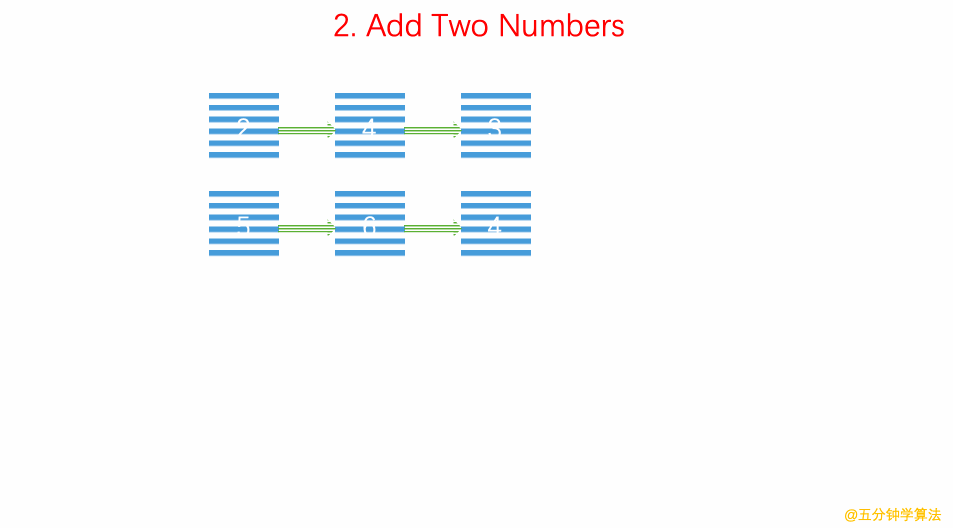### 题目描述

``````输入：(2 -> 4 -> 3) + (5 -> 6 -> 4)

``````

### 动画描述### 代码实现

``````/// 时间复杂度: O(n)
/// 空间复杂度: O(n)
/**
* public class ListNode {
*     int val;
*     ListNode next;
*     ListNode(int x) { val = x; }
* }
*/
class Solution {
public:
ListNode* addTwoNumbers(ListNode* l1, ListNode* l2) {

ListNode *p1 = l1, *p2 = l2;
int carried = 0;
while(p1 || p2 ){
int a = p1 ? p1->val : 0;
int b = p2 ? p2->val : 0;
cur->next = new ListNode((a + b + carried) % 10);
carried = (a + b + carried) / 10;

cur = cur->next;
p1 = p1 ? p1->next : NULL;
p2 = p2 ? p2->next : NULL;
}

cur->next = carried ? new ListNode(1) : NULL;